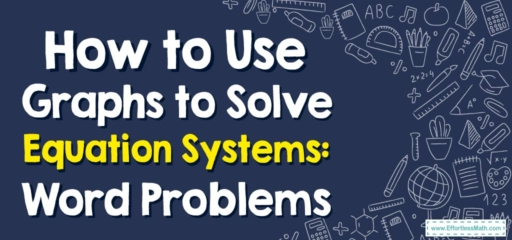# How to Use Graphs to Solve Equation Systems: Word Problems

In the mathematical universe, graphs and equation systems converge in harmony, guiding us to elegant solutions for complex problems. The merger of these mathematical concepts provides a robust method for tackling a variety of word problems. This article will elucidate a step-by-step approach to utilizing graphs to solve equation systems in word problems.## A Step-by-step Guide to Using Graphs to Solve Equation Systems: Word Problems

Equation systems represent a collection of two or more equations with a similar set of variables. Graphs, on the other hand, visualize equations by representing them in the Cartesian plane. This combination presents a compelling method to interpret and solve a multitude of real-life situations, neatly encapsulated in word problems.

### Step 1: Understanding the Word Problem

Every word problem narrates a scenario requiring a mathematical solution. Here, you must identify the unknown variables and frame equations representing the given situation. For instance, let’s consider a problem relating two different taxi services with their fare structures given. The unknowns here could be the distance covered or the total fare, giving us two linear equations.

### Step 2: Formulating the Equation System

The next step involves translating the word problem into an equation system. The equations should correspond to the relationships or conditions described in the problem. With our taxi services example, we might generate equations like $$y=3x+2$$ and $$y=4x+1$$ where $$y$$ represents the total fare, $$x$$ the distance and the constants are based on the fare structures given.

### Step 3: Graphing the Equations

With the equation system in place, the next course of action is to draw the graph for each equation. You plot each equation on the same Cartesian plane. This visual representation effectively demonstrates the relationship between the variables in a dynamic way that numbers alone cannot.

### Step 4: Identifying the Solution

The point where the two graphs intersect is the solution to the system. This intersection signifies the value of the variables that satisfy both equations simultaneously. In our taxi services scenario, this point represents the distance at which both services charge the same fare.

### Step 5: Verifying the Solution

A key step in mathematics is always to verify your solution. Plug the obtained solution back into the original equations to ensure they hold true. If they do, you’ve found the correct solution. If not, revisit your calculations.

### What people say about "How to Use Graphs to Solve Equation Systems: Word Problems - Effortless Math: We Help Students Learn to LOVE Mathematics"?

No one replied yet.

X
30% OFF

Limited time only!

Save Over 30%

SAVE $5 It was$16.99 now it is \$11.99